## Equation in Word 365 2016 2013 2010 2007 2003

### How to create a cross-reference to an equation in Word

You can create two types of numbering for formulas and equations in the Word document:

### How to create vectors in Word

A vector is a mathematical object characterized by magnitude and direction. To define a vector, you can use:
• a bar above (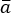) - see how to work with Superimposing characters,
• an arrow above (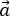),
• bold or gothic font (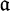) - see how to insert Double strike or Blackboard bold symbols and gothic symbols in Word,
• a letter with a tilde (~) underneath it (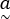) or (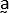), see below how to create such vectors.

### How to create captions for equations

When you add formulas to your document, it may need to to add captions - right-justified automatic numbering on the same line as equation. It is easy to add captions for the equation:

### Working with Microsoft Equation

An equation is a mathematical statement that two mathematical expressions are equal (indicated by the sign =). Microsoft Equation helps you type fractions, exponents, integrals, and so on in Word documents. In addition, Word allows you to insert an equation from the Gallery, create a new equation, edit, change, or delete a part or the entire equation.

### Insert a vertical bar with integration limits into the equation

For some standards, it is necessary to add to the equation the vertical bar with integration limits. Microsoft Word doesn't propose the simple way how to add it.

### How to insert an equation with a system of linear equations or linear system

This tip shows how to add an equation with a system of linear equations (or linear system) – a collection of two or more linear equations involving the same set of variables.

### How to insert an equation with trigonometric functions

This tip shows how to add an equation with trigonometric functions, for example, the Euler's formula.

### How to insert an equation with a limit

This tip shows how to add an equation with a limit. For example, the formula of a limit.

### How to insert a Boolean algebra equation

This tip shows how to insert a Boolean algebra equation, for example, one of De Morgan's laws.

### How to quickly insert Script symbols in Word equations

In mathematics often is used a script Latin alphabet. This tip is about quick way how to paste letters in Script in your Microsoft Word document.

We use cookies to personalise content and ads, to provide social media features and to analyse our traffic. We also share information about your use of our site with our social media, advertising and analytics partners who may combine it with other information you’ve provided to them or they’ve collected from your use of their services.